##### PostgreSQL ROUND() Function With Examples

Rounding off a number up to specific decimal places is a very common task. PostgreSQL offers a ROUND() function that takes either one or two arguments/values. If the ROUND() function takes only one value, then it will skip the fractional part and round the given number to the nearest integral value.

On the other hand, if the ROUND() function takes two parameters/values, then the second parameter specifies the number of decimal places. For example, if the second parameter’s value is 2, then the given value will be rounded off to two decimal places.

This write-up will present detailed knowledge about the ROUND() function with the help of examples. So, let’s begin.

How to Use ROUND() Function in PostgreSQL?

To avail the functionalities of the ROUND() function, you have to follow the below syntax:

`ROUND(Number [ , n]);`

Here, in this syntax, the “Number” represents a numeric value to be rounded. While “n” is an optional parameter that determines the number of decimal points.

Example #1: How to Use the ROUND() Function in PostgreSQL?

This example illustrates the working of the ROUND() function that accepts only one value/parameter:

`SELECT ROUND(72.125);`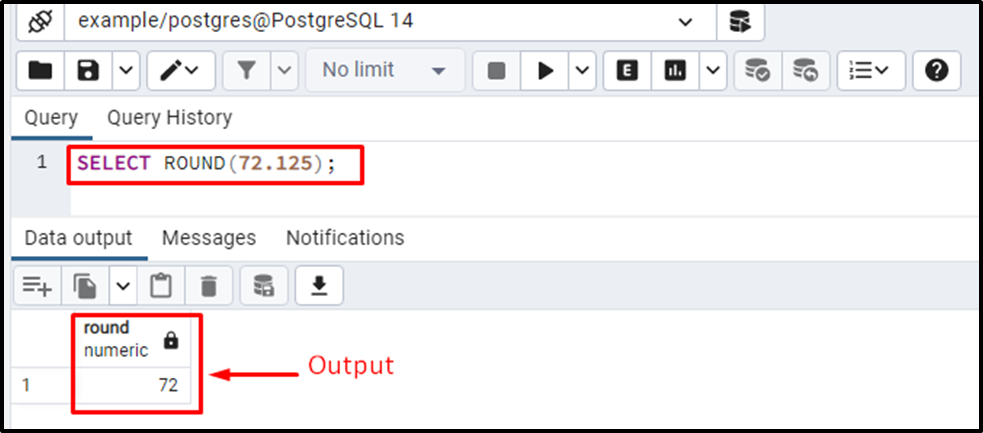The output verifies that the ROUND() function successfully rounded the given number to the nearest integral value. The fractional value was less than 5, so the given number was rounded downward.

Example #2: How to Round a Number to an Integer Using ROUND() Function?

Let’s take another example of the ROUND() function that accepts only one parameter to understand its working in a better way:

`SELECT ROUND(72.725);`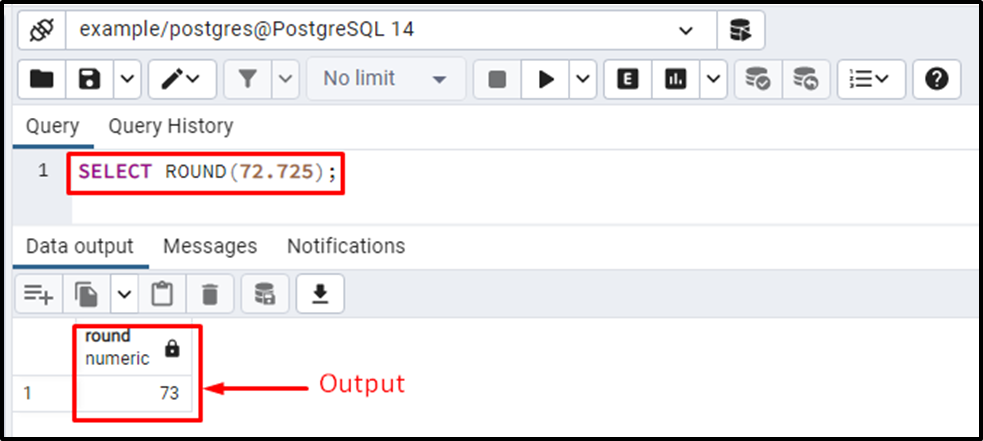This time, the fractional value was greater than 5 (i.e., .725). Therefore the ROUND() function rounded the given number upwards, i.e., 73.

Example #3: How to Round a Numeric Value up to Two Decimal Points?

The task is to round the given number up to two decimal places, so we will pass two parameters to the ROUND() function:

`SELECT ROUND(72.1214, 2);`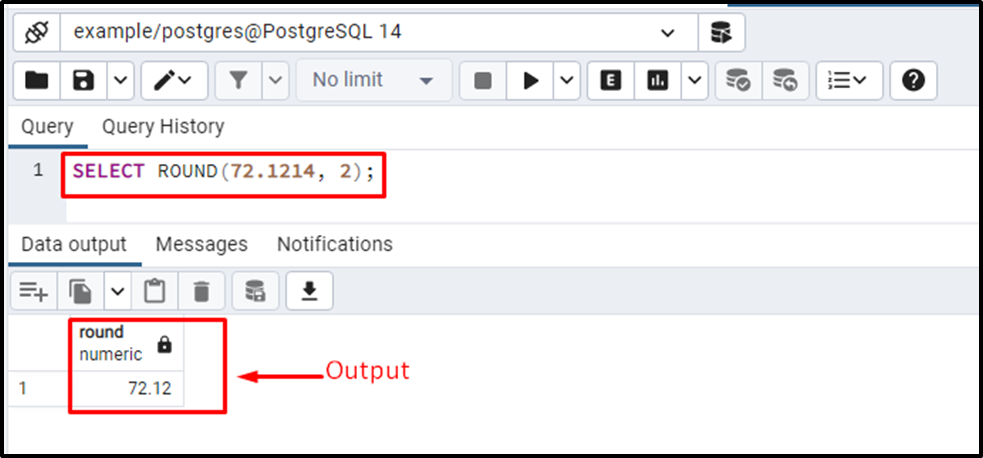The output verifies that the given number has been rounded up to two decimal places. Let’s consider another example for a profound understanding of the ROUND() function.

Example #4: How to Round a Numeric Value up to Three Decimal Points?

Let’s pass ‘3’ as a second parameter to the ROUND() function to get a number rounded up to three decimal places:

`SELECT ROUND(72.12765, 3);`

- In this example, the original number is “72.12765”, the task is to round the given number up to three places.

- The fourth number after the decimal is 6, which is greater than 5.

- So the ROUND() function will not only round the given number up to 3 places but also round the third place digit upward i.e., “.127” will be rounded to “.128”: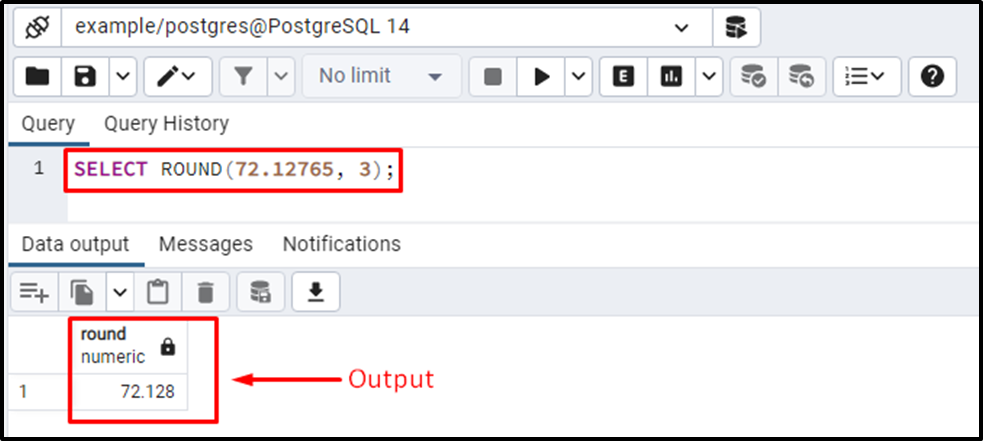The output authenticates the working of the ROUND() function.

How to Round the Table’s Data Using the ROUND() Function?

The ROUND() function is equally effective in rounding the table’s data. Suppose we have a table staff_details whose details are as follows:

`SELECT * FROM staff_details;`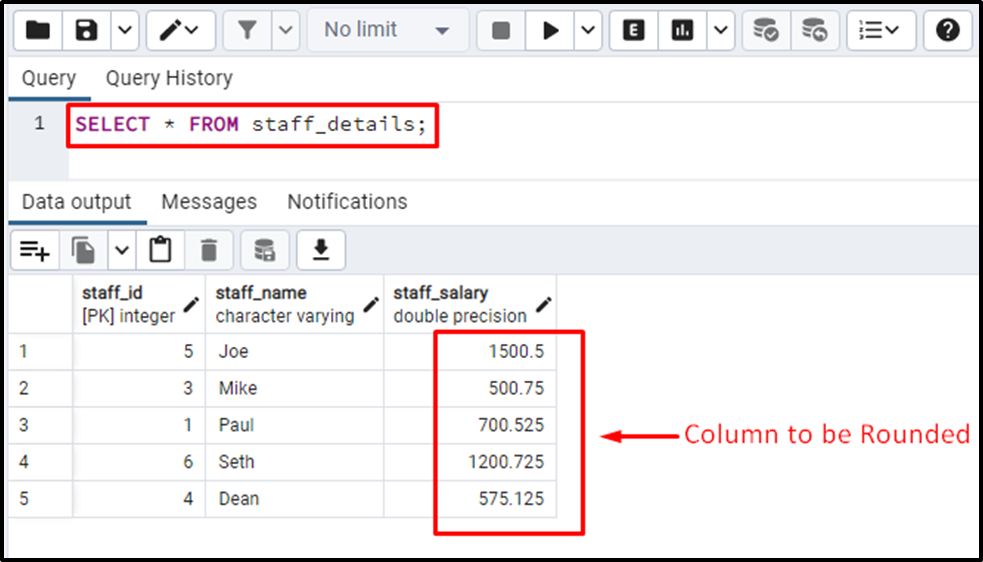Let’s say we have to round all the values of a column “staff_salary”. To do that, execute the below query:

```SELECT staff_name,
ROUND(staff_salary)
FROM staff_details;```

The above query will fetch the staff_name and staff_salary from the staff_details table. The ROUND() function is applied to the staff_salary column. Therefore the salary will be rounded to the nearest integral value: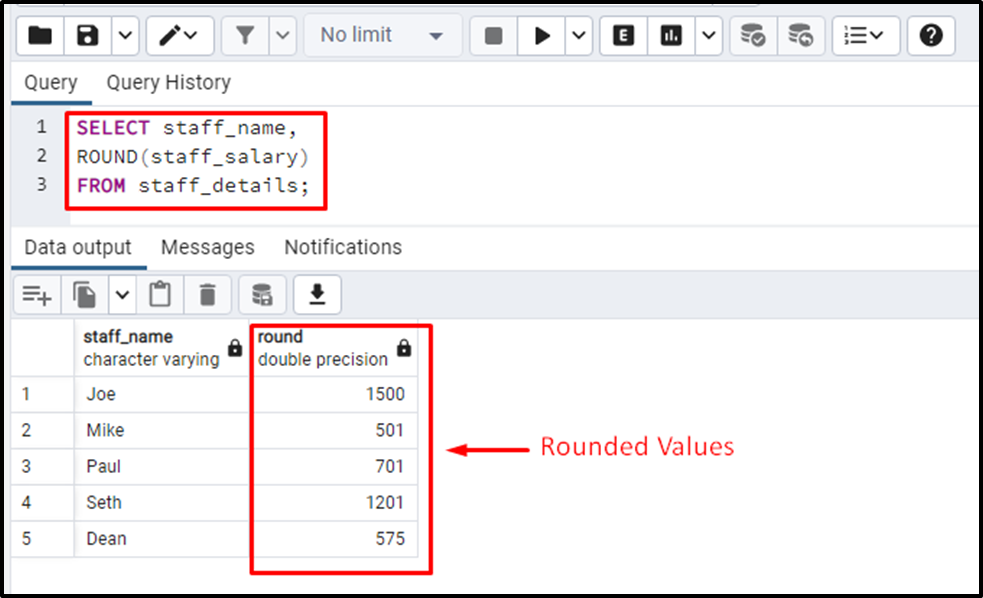The output shows that the ROUND() function succeeded in rounding the values of the staff_salary column.

Conclusion

PostgreSQL provides a built-in function named the ROUND() function that accepts either one or two arguments/values. If the ROUND() function accepts only one argument/value, then it will skip the fractional part and round the given number to the nearest integral value. Else if the ROUND() function accepts two arguments/values, then the second parameter specifies the number of decimal places up to which the given value will be rounded. This write-up explained how the ROUND() function works in PostgreSQL using some examples.